# Understanding Undefined Behaviour in C

0
3719In the first part of this article, we discussed sequence points in C as well as case studies of undefined behaviour, using various examples like modifying the string literal and signed integer overflow. The second part of the article discusses a few more such case studies.

Let’s now look at a few more examples like integer division by zero, oversized shift operations, and dereferencing the null pointer and array out of bounds —all of which lead to undefined behaviour.

Case study 4: Dividing an integer by zero is undefined behaviour

According to c99 standards (§6.5.5/5): “The result of the / operator is the quotient from the division of the first operand by the second; the result of the % operator is the remainder. In both operations, if the value of the second operand is zero, the behaviour is undefined.”

Instance 1:

```1 #include <stdio.h>

2

3 int main()

4 {

5 int x = 0;

6 double y = 10 / x;

7 /* Some code here */

8 return 0;

9 }```

In program-6 above, line #6 ends up in undefined behaviour since the value 10 is divided by zero. The code executed using the gcc compiler results in the runtime error being called ‘floating point exception’ (core dumped). The same has been shown in Figure 1.Figure 1: Output of program-6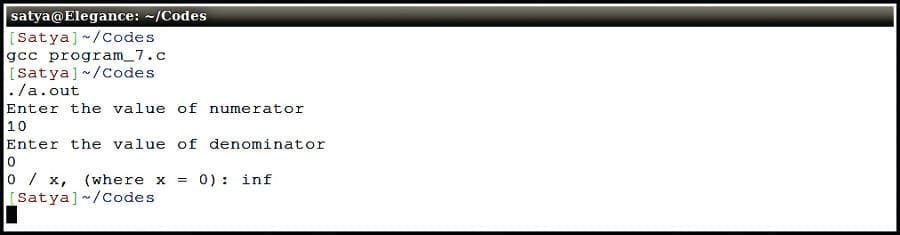Figure 2a: When the numerator is positiveFigure 2b: When the numerator is negative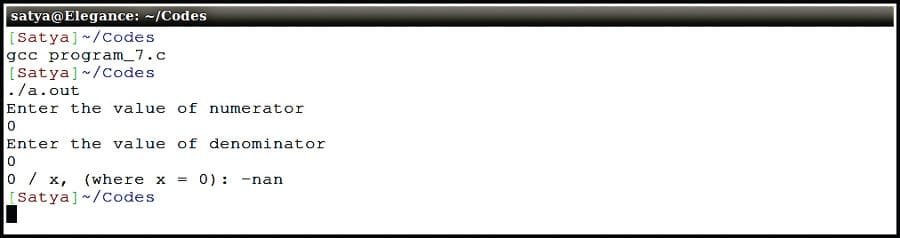Figure 2c: When the numerator is zero

Instance 2: But for floating point numbers (as per the IEEE standards), division of a finite non-zero float value by 0 is ‘well-defined’ and results in +infinity (+INF) or -infinity (-INF) according to the sign of the first operand. The result of x/0 for x=0 gives NaN (Not-a-Number).

```1 #include <stdio.h>

2

3 int main()

4 {

5 double denominator, numerator;

6

7

8 /* Prompt the user to enter the value of numerator */

9 printf(“Enter the value of numerator\n”);

10

11 /* Read the value */

12 scanf(“%lf”, &numerator);

13

14 /* Prompt the user to enter the value of denominator */

15 printf(“Enter the value of denominator\n”);

16

17 /* Read the value */

18 scanf(“%lf”, &denominator);

19

20 /* Read the value */

21 double y = numerator / denominator;

22

23 printf(“0 / x, (where x = 0): %lf\n”, y);

24

25 return 0;

26 }```

In program-7 above, x is a double; hence, 10/x gives +inf as the output when the gcc compiler is used. Similarly, -10/x will yield the result -inf and, finally, 0/0 will give NaN. The output is shown in Figures 2a, 2b and 2c. Since most of the C language conforms to the IEEE floating-point standards and the latest versions of the gcc compiler also support it, division by zero yields well-defined behaviour.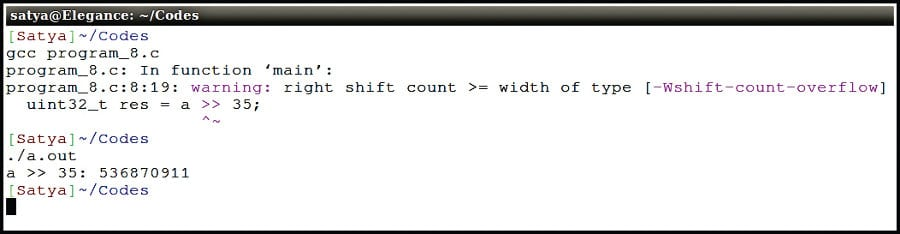Figure 3: Output of program-8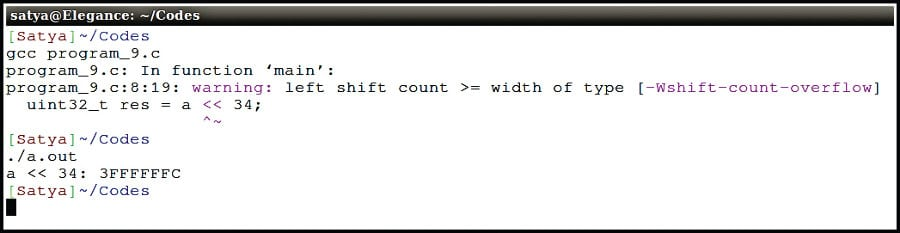Figure 4: Output of program-9

Case study 5: Oversized shift operation is undefined behaviour

The C standard says, “The integer promotions are performed on each of the operands. The type of the result is that of the promoted left operand. If the value of the right operand is negative or is greater than or equal to the width of the promoted left operand, the behaviour is undefined.”

Instance 1: Consider the demo code shown in program-8.

```1 #include <stdio.h>

2 #include <stdint.h>

3

4 int main()

5 {

6 uint32_t a = 0xFFFFFFFF;

7

8 uint32_t res = a >> 35;

9

10 printf(“a >> 35: %u\n”, res);

11

12 return 0;

13 }```

In line #8, the value of the variable is shifted to the right n times, where n is 0<=n<=MAX_BITS. The resultant value will be the integral part of the quotient of ‘value / (2^n)’. But, from program-8, the value of the variable a is shifted right by more than the maximum number of bits allowed in an integer (in this case, sizeof(int) is assumed to be 4 bytes, i.e., 32 bits). So the result will be undefined behaviour according to the c99 standard.

The output of program-8 is shown in Figure 3.

From the output shown in Figure 3, you can see the gcc compiler generates a warning upon executing the code, as the result is some garbage value instead of zero.

Instance 2: According to the c99 standard, “The result of E1 << E2 is E1 left-shifted E2 bit positions; vacated bits are filled by zeros. If E1 has an unsigned type, the value of the result is E1 × 2E2, reduced modulo one more than the maximum value representable in the result type. If E1 has a signed type and non-negative value and E1 × 2E2 is representable in the result type, then that is the resulting value; otherwise, the behaviour is undefined.”

Consider the demo code shown in program-9 below:

```1 #include <stdio.h>

2 #include <stdint.h>

3

4 int main()

5 {

6 int32_t a = 0x0FFFFFFF;

7

8 int32_t res = a << 34;

9

10 printf(“a << 34: %X\n”, res);

11

12 return 0;

13 }

```

In line #8, the value of the variable is shifted to the left n times, where n is 0<=n<=MAX_BITS. The resultant value will be the integral part of the quotient of ‘value * (2^n)’. But, from program-8, the value of the variable a is shifted to the left by more than the maximum number of bits allowed in an integer (in this case, sizeof(int) is assumed to be 4 bytes, i.e., 32 bits). So the result will be undefined behaviour as given in the c99 standard documentation.

The output of program-9 is shown in Figure 4.

Similarly, when the variable value is left-shifted or right-shifted by a negative number, the result will be undefined as shown in program-10 below. The output is shown in Figure 5.

```1 #include <stdio.h>

2 #include <stdint.h>

3

4 int main()

5 {

6 uint32_t a = 0x0FFFFFFF;

7

8 uint32_t res = a << -2;

9

10 printf(“a << 34: %X\n”, res);

11

12 return 0;

13 }```

A summary of case study 5, considering the E1 bit-wise left/right shift by E2, where E1 and E2 are left and right operands respectively, is given in Table 1.Case study 6: Differencing the null pointer is undefined behaviour

Null is a macro whose implementation is defined as a null pointer constant. According to the c99 standard, Null is defined as integer value 0, which is implicitly or explicitly type-casted to void*.

The c99 section 6.5.3.2, 4th point, says, “The unary * operator denotes indirection. If the operand points to a function, the result is a function designator; if it points to an object, the result is an lvalue designating the object. If the operand has type ‘pointer to type’, the result has type ‘type’. If an invalid value has been assigned to the pointer, the behaviour of the unary * operator is undefined.”

The literal meaning of the null pointer is: “Not pointing anywhere.” But dereferencing it leads to undefined behaviour in C, which means anything can happen, i.e., the program may crash, or be transformed into an exception that can be caught or even silently run the code, leading to some strange result every time the code is executed. When concerned with embedded systems, hardware must either detect access to location zero and restart the system, or access to location zero can be made by having the C compiler insert code to check it each time. But this simply adds an extra burden for the C compiler, which finally increases the time and space complexity.

There are certain situations where dereferencing the null pointer is perfectly valid and does not lead to a program crash, as in the case of a UNIX-like OS, but is intentionally well-defined. In a Linux-like OS, however, dereferencing the null pointer always yields the consistent result ‘segmentation fault’.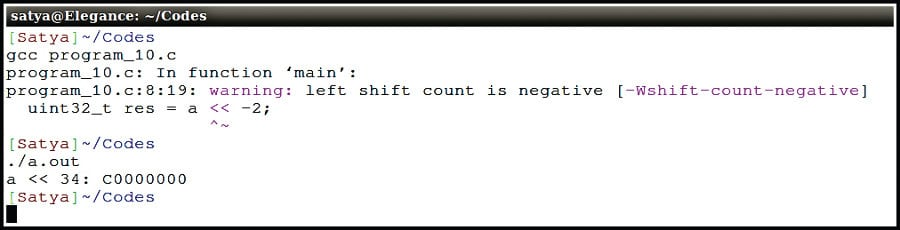Figure 5: Output of program-10

Consider the sample demo code shown in program-11 below:

```1 #include <stdio.h>

2

3 int main()

4 {

5 int *p;

6

7 p = NULL;

8

9 /*Some code here*/

10

11 /* Dereferencing the NULL pointer */

12 *p = 10;

13

14 /* Some code here */

15

16 return 0;

17 }```

This code is compiled using the gcc compiler and executed under the Linux OS run on an Intel processor. In line #12, dereferencing the null pointer (p) makes the kernel send the signal (SIGSEGV) to the process, since the page fault handler fails to handle this.

The output of program-11 is shown in Figure 6.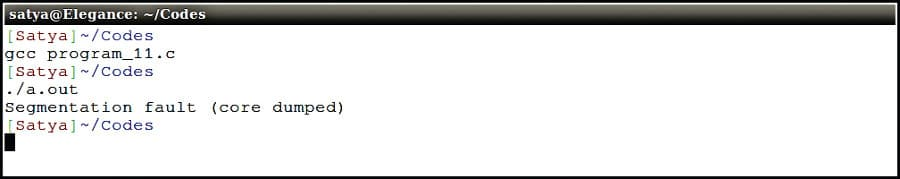Figure 6: Output of program-11Figure 7: Assembly code of program-11

Figure 7 shows the assembly code generated for program-11, which clearly indicates that the gcc compiler is silently allowing dereferencing the null pointer and is also not generating any extra code to make an attempt to check the null pointer dereferencing. Finally, it is all up to the Linux OS to handle this.

Case study 7: Array—out of bounds is undefined behaviour

Accessing elements beyond the boundary of the array limit is undefined behaviour in the C programming language according to the C standards.

Unlike some other languages like Java, checking of array bounds is not present in the C language; it is all up to the programmer to take care of it.

Let us understand this through a demo code shown in program-12 below:

```1 #include <stdio.h>

2 #define MAX 5

3

4 int main()

5 {

6 //Define an array of size MAX

7 int a[MAX] = {1, 2, 3, 4, 5};

8

9 //Access the item present at the index 5

10 printf(“a: %d\n”, a);

11

12 return 0;

13 }```

In line #10, we are printing the element that is present at index 5, which is out of boundary for the given array. The C standard says that accessing the items beyond the array limit is undefined behaviour. This means that anything can happen, and one can either end up with a system crash in case of non-OS based embedded systems, or with segmentation fault (commonly) in case of OS based embedded systems or OS based non-embedded systems.

Let us consider two different scenarios, with reference to program-13 given below.

```1 #include <stdio.h>

2 #define MAX 5

3

4 int main()

5 {

6 //Define an array of size MAX

7 int a[MAX] = {1, 2, 3, 4, 5};

8

9 //Assigning the value to the array location present at index 5

10 a = 6;

11

12 //Access the item present at the index 5

13 printf(“a: %d\n”, a);

14

15 return 0;

16 }```

Scenario #1– non-OS based embedded systems: Assuming program-13 is run under a non-OS based embedded system, in line #10 there is a statement which accesses the array location beyond the array limitation. At this location, if some critical information is present, then the statement present in line #10 overwrites it, which leads to losing the critical information and also ending up with a system crash.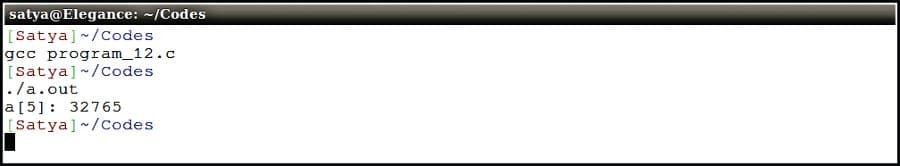Figure 8: Output of program-12Figure 9: Output of program-13

Scenario #2—OS based embedded systems and non-embedded systems: If the same program-13 is run under OS based embedded systems and non-embedded systems, any one of the following things may happen:

1. Segmentation fault, if the 5th index falls out of process space. This only happens when you try to read or write to a page that was not allocated by the OS, or try to do something on a page that is not permitted (e.g., try to write to a read-only page); since pages are usually big (often in multiples of a few KBs), this ends up with room to overflow.

2. Critical information is overwritten, if present.

3. If memory space is free beyond the array limit, it simply goes and writes to it which leads to the buffer overflow.

4. Program may terminate abruptly.

5. Any strange unexpected thing can happen.

The output of program-12, compiled and run under the Intel based Linux platform with gcc compiler, is shown in Figure 8. It silently compiles without giving any warning signs even if the -Wall option is enabled during the compilation. In our case, we get the result as the garbage value present at the index 5.

The output of program-13 is shown in Figure 9. Since we are running the code under the OS based non-embedded system, we are ending up with the buffer overflow situation.

From the illustrations given in program-12 and program-13, it is clear that accessing the array items beyond the array limits leads to undefined behaviour. So it is always better to use a static or dynamic analyser to analyse the problems, such as the PVS-Studio static analyser, which is an open source tool.

Advertisement
SHAREThe author is a member of the embedded software team at Emertxe Information Technologies (http://www.emertxe.com). His area of interest lies in embedded C programming combined with data structures and microcontrollers. He likes to experiment with C programming in his spare time to explore new horizons. He can be reached at satya@emertxe.com